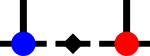SyTensyten::STensorImpl::Autodiff Namespace Reference

Contains classes and functions to deal with autodifferentiation of STensor objects. More...

## Classes

class  ComputeNode
Describes an individual function call as part of a computation. More...

## Typedefs

using AdjointEvaluator = std::function< STensor(ComputeNode &node, Size input_number, STensorId const result_id)>
Type of the input adjoint evaluator which can access the adjoints of all output values as well as the stored input and output tensors of the node to evaluate an output adjoint of an upstream node. More...

using ComputeNodePtr = std::shared_ptr< ComputeNode >
Type of a shared pointer to a compute node. More...

using ComputeNodeWPtr = std::weak_ptr< ComputeNode >
Type of a weak pointer to a compute node, used for the "downstream" direction. More...

using STensorId = std::uint64_t
Randomly-generated ID of an STensor, to be used during automatic differentiation. More...

## Functions

ComputeNodePtr create (std::string &&opname_, Vec< Pair< ComputeNodePtr, Size > > &&input_nodes_, Vec< STensorId > &&output_ids_, AdjointEvaluator &&func_, Vec< STensor > &&output_shapes_, Vec< AsyncCached< STensor > > &&cached_tensors_={})
Helper to create a new ComputeNode and return a shared pointer to it. More...

ComputeNodePtr create_primer (ComputeNodePtr input_node_ptr, Size input_node_number, STensorId output_id, std::int8_t value, SBasis basis_on_tensor, STensor const &shapelike)
Helper to create a priming ComputeNode and a return a shared pointer to it. More...

void create_product_differentiable (STensorProxy const &a, STensorProxy const &b, STensor &return_value)
Sets the compute nodes of the tensor return_value to represent the product of tensor proxies a and b. More...

STensorId new_id ()
Generates a new tensor ID larger than 999 to be used by auto-generated tensors. More...

STensor qr_adjoint_evaluator (ComputeNode &node, STensorId const result_id, SBasisId const &int_leg)
Evaluates the adjoint of a QR decomposition identified by ComputeNode node. More...

STensor return_first_output_adjoint (ComputeNode &node, Size input_number, STensorId const result_id)
AdjointEvaluator-typed function which simply returns the output adjoint of the first output. More...

STensor svd_adjoint_evaluator (ComputeNode &node, STensorId const result_id, SBasisId const &us_basis, SBasisId const &sv_basis)
Evaluates the adjoint of a SVD identified by ComputeNode node. More...

## Detailed Description

Contains classes and functions to deal with autodifferentiation of STensor objects.

Autodifferentiation is a method to automatically calculate exact derivatives of functions at specific values. This is done by tracing along a calculation and storing all data necessary to evaluate partial derivates. Later, the derivative can be obtained by walking backwards in this history and evaluating the individual partial derivates, accumulating them to a total derivative.

Here, this is implemented in three parts:

• each STensor can optionally store a shared pointer to a ComputeNode object (and an ID which uniquely identifies this result). This storage is enabled when STensor::autodiff_enabled() returns true and can be enabled by e.g. calling STensor::enable_autodiff() on the tensor.
• each ComputeNode object represents a particular instantiation of a function call. That is, it knows which tensors (or other constants) were used as inputs and which tensors were produced as outputs. It also stores a pointer to a function which can be evaluated to (back)propagate the derivative through this compute node. This ComputeNode object must be updated on each action taken on the tensor. Hence, functions which change or manipulate tensors should either assert that autodifferentiation is disabled for their inputs (which means their outputs don't need it either) or should appropriately adapt the compute nodes of the output tensors. Care must be taken not to accidentally delete shared pointers to other compute nodes in the process, as this would result in those nodes potentially being deleted as well. For examples on how to do this, see the numerous files in this subdirectory.
• When a derivative of a result tensor with respect to an input tensor is actually requested, the history is walked backwards and double-linked to also include links from upstream compute nodes to downstream compute nodes. The function the requests the output adjoint (i.e., the derivative) of the original node which produced the input tensor as an output. This node then recursively requests the output adjoints of all their child nodes which are in turn recursively evaluated.

Multiple caveats apply here:

• At the moment, we assume that conj creates a new, independent, tensor. This is true for complex conjugations, as it is not possible to differentiate through a complex conjugation, but it is not true if the calculation uses real numbers only, as then conj is more similar to a transpose function. Representing the derivative of such a transpose function, however, is quite annoying.
• There are some tensor networks which are valid to contract but whose derivatives cannot be represented in the STensor formalism. For example, given two tensors A[i,j] and B[j,i1] the product A[i,j]·B[j,i1] = C[i,i1] is well-defined. We can then also further prime the second index and afterwards the first to give C[i1,i2]. However, now differentiating this object with respect to B[j,i1] would create a new tensor with four open indices: i1, i2 from C, j from A and a new i1 which belongs to a vitual δ[i1,i1] tensor used to prime the old index of B. As a result, this tensor would have two legs with identical indices. This problem mostly occurs with ‘interestingly’ primed tensor legs and taking derivatives of not fully contracted tensor networks.
• There are certainly some functions which do not assert disabled autodifferentiability yet but also don't setup the compute nodes properly.

To use:

• Enable autodifferentiation in your input tensor at the start of your calculation using STensor::enable_autodiff(x) where x is a number between 1 and 999 inclusive.
• Once the result is obtained, call STensor::autodiff(x) on it.
• Double-check that everything is correct by calling STensor::get_autodiff_node()->draw(std::cout) on the result. This will cause a dot-compatible compute graph to be printed to the supplied stream. In the graph, nodes are compute nodes, edges are outputs from one node being used in another. Those outputs are labelled by numbers starting at 1000.